# Basic polymer simulations in ESPResSo¶

In this tutorial we are going to investigate diffusion of a dissolved polymer using ESPResSo. For this tutorial, you should have fundamental knowledge of the Lattice-boltzmann method and Langevin dynamics. If you are unfamiliar with those, you can go through the respective tutorials in the lattice_boltzmann and langevin_dynamics folders.

## Introduction¶

In these exercises we want to reproduce a classic result of polymer physics: the dependence of the diffusion coefficient of a polymer on its chain length. If no hydrodynamic interactions are present, one expects a scaling law $D \propto N ^{- 1}$ and if they are present, a scaling law $D \propto N^{- \nu}$ is expected. Here $\nu$ is the Flory exponent that plays a very prominent role in polymer physics. It has a value of $\sim 3/5$ in good solvent conditions in 3D. Discussions on these scaling laws can be found in polymer physics textbooks like , , and [3, chapter 8].

The reason for the different scaling law is the following: when being transported, every monomer creates a flow field that follows the direction of its motion. This flow field makes it easier for other monomers to follow its motion. This makes a polymer (given it is sufficiently long) diffuse more like a compact object including the fluid inside it, although it does not have clear boundaries. It can be shown that its motion can be described by its hydrodynamic radius. It is defined as:

\begin{equation} \left\langle \frac{1}{R_h} \right\rangle = \left\langle \frac{1}{N^2}\sum_{i\neq j} \frac{1}{\left| r_i - r_j \right|} \right\rangle \end{equation}

This hydrodynamic radius exhibits the scaling law $R_h \propto N^{\nu}$ and the diffusion coefficient of a long polymer is proportional to its inverse $R_h$.

## Polymer models¶

The diffusion coefficient $D$ of a spherical particle in a carrier fluid experiencing drag is related to the friction coefficient $\zeta$ via the Einstein relation:

\begin{equation} D = \frac{k_{\mathrm{B}}T}{\zeta}, \end{equation}

with $k_{\mathrm{B}}$ the Boltzmann constant and $T$ the temperature. For a sphere of radius $R$ moving in a fluid of viscosity $\eta$, the friction coefficient is obtained via the Stokes law:

\begin{equation} \zeta = 6\pi\eta R. \end{equation}

Combining both equations yields the Stokes–Einstein relation:

\begin{equation} D = \frac{k_{\mathrm{B}}T}{6\pi\eta R}. \end{equation}

The simplest description of a polymer is the Rouse model, where beads are connected by springs. All beads experience a drag from the solvent, and the friction coefficient $\gamma$ is identical for all beads. The solvent flows freely between beads and hydrodynamic interactions are neglected. The diffusion coefficient takes the following form:

\begin{equation} D_{\mathrm{R}} = \frac{D_0}{N} = \frac{k_{\mathrm{B}}T}{\gamma N}, \end{equation}

where $D_0$ is the diffusion coefficient of a single bead.

To account for hydrodynamic interactions mediated by the solvent, i.e. the transport of solvent in contact with the beads and the correlation in the motion of beads due to the carried solvent, the Zimm model was created. For an ideal chain, it takes the following form:

\begin{equation} D_{\mathrm{Z}} = \frac{8}{3\sqrt{6\pi^3}}\frac{k_B T}{\eta R} \simeq 0.196\frac{k_B T}{\eta b N^{\nu}}, \end{equation}

with $R$ the radius of the polymer and $b$ the length of the spring connecting the beads.

For shorter polymers there is a transition region. It can be described by the Kirkwood–Zimm model:

\begin{equation} D=\frac{D_0}{N} + \frac{k_B T}{6 \pi \eta } \left\langle \frac{1}{R_h} \right\rangle \end{equation}

Here $D_0$ is the monomer diffusion coefficient and $\eta$ the viscosity of the fluid. For a finite system size the second part of the diffusion is subject to a $1/L$ finite size effect, because hydrodynamic interactions are proportional to the inverse distance and thus long ranged. It can be taken into account by a correction:

\begin{equation} D=\frac{D_0}{N} + \frac{k_B T}{6 \pi \eta } \left\langle \frac{1}{R_h} \right\rangle \left( 1- \left\langle\frac{R_h}{L} \right\rangle \right) \end{equation}

It is quite difficult to fit this analytical expression to simulation data with good accuracy. It will need a LB fluid, long simulation times and a careful analysis. For this tutorial, we will use an implicit solvent and short polymer lengths to keep the runtime short. If you want to collect data suitable for the Zimm model, simply set the global variable POLYMER_MODEL to 'Zimm', and increase the box size and number of beads in the polymer.

We want to determine the long-time self diffusion coefficient from the mean square displacement of the center-of-mass of a single polymer. For large $t$ the mean square displacement is proportional to the time and the diffusion coefficient occurs as a prefactor:

\begin{equation} D = \lim_{t\to\infty}\left[ \frac{1}{6t} \left\langle \left(\vec{r}(t) - \vec{r}(0)\right)^2 \right\rangle \right]. \end{equation}

This equation can be found in virtually any simulation textbook, like . We will set up a polymer in an implicit solvent, simulate for an appropriate amount of time, calculate the mean square displacement as a function of time and obtain the diffusion coefficient from a linear fit. However we will have a couple of steps inbetween and divide the full problem into subproblems that allow to (hopefully) fully understand the process.

## Diffusion of a polymer¶

One of the typical applications of ESPResSo is the simulation of polymer chains with a bead-spring-model. For this we need a repulsive interaction between all beads, for which one usually takes a shifted and truncated Lennard-Jones (so-called Weeks–Chandler–Andersen or WCA) interaction, and additionally a bonded interaction between adjacent beads to hold the polymer together. You have already learned that the command

system.non_bonded_inter[0, 0].lennard_jones.set_params(
epsilon=1.0, sigma=1.0, shift=0.25, cutoff=1.1225)


creates a Lennard-Jones interaction with $\varepsilon=1.$, $\sigma=1.$, $r_\text{cut} = 1.1225$ and $\varepsilon_\text{shift}=0.25$ between particles of type 0, which is the desired repulsive interaction. The command

fene = espressomd.interactions.FeneBond(k=7, r_0=1, d_r_max=2)


creates a FeneBond object (see ESPResSo manual for the details). What is left to be done is to add this bonded interaction to the system via

system.bonded_inter.add(fene)


and to apply the bonded interaction to all monomer pairs of the polymer as shown in the script below.

ESPResSo provides a function that tries to find monomer positions that minimize the overlap between monomers of a chain, e.g.:

positions = espressomd.polymer.linear_polymer_positions(n_polymers=1,
bond_length=1, seed=42,
min_distance=0.9)


which would create positions for a single polymer with 10 monomers. Please check the documentation for a more detailed description.

### 1. Setting up the polymer and observables¶

The first task is to compute the average hydrodynamic radius $R_h$, end-to-end distance $R_F$ and radius of gyration $R_g$ for different polymer lengths. This will be achieved with the corresponding observables described in the user guide under Analysis / Direct analysis routines / Chains.

The second task is to estimate the polymer diffusion coefficient for different polymer lengths using two methods:

• the center of mass mean squared displacement method (introduced in a previous part of this tutorial)
• the center of mass velocity autocorrelation method (also known as Green–Kubo method)

For this purpose we can again use the multiple tau correlator.

Write a function with signature build_polymer(system, n_monomers, polymer_params, fene) that creates a linear polymer made of n_monomers particles, with parameters polymer_params. The particles need to be created and linked together with the fene bond.

In :
def build_polymer(system, n_monomers, polymer_params, fene):
positions = espressomd.polymer.linear_polymer_positions(
p_previous = None
for i, pos in enumerate(positions):
if p_previous is not None:
p_previous = p


Write a function with signature correlator_msd(pids_monomers, tau_max) that returns a center-of-mass mean-squared displacement correlator that is updated every time step, and a function with signature correlator_gk(pids_monomers, tau_max) that returns a center-of-mass velocity correlator that is updated every 10 time steps. You can find examples in the user guide section calculating a particle's diffusion coefficient.

In :
def correlator_msd(pids_monomers, tau_max):
com_pos = espressomd.observables.ComPosition(ids=pids_monomers)
com_pos_cor = espressomd.accumulators.Correlator(
obs1=com_pos, tau_lin=16, tau_max=tau_max, delta_N=5,
return com_pos_cor

def correlator_gk(pids_monomers, tau_max):
com_vel = espressomd.observables.ComVelocity(ids=pids_monomers)
com_vel_cor = espressomd.accumulators.Correlator(
obs1=com_vel, tau_lin=16, tau_max=tau_max, delta_N=10,
return com_vel_cor


You can simulate a polymer in the Rouse regime using an implicit solvent model, e.g. Langevin dynamics, or in the Zimm regime using a lattice-Boltzmann fluid.

In :
def solvent_langevin(system, kT, gamma):
'''
Implicit solvation model based on Langevin dynamics (Rouse model).
'''
system.thermostat.set_langevin(kT=kT, gamma=gamma, seed=42)

def solvent_lbm(system, kT, gamma):
'''
Lattice-based solvation model based on the LBM (Zimm model).
'''
lbf = espressomd.lb.LBFluidGPU(kT=kT, seed=42, agrid=1, dens=1,
visc=5, tau=system.time_step)
system.thermostat.set_lb(LB_fluid=lbf, gamma=gamma, seed=42)


### 2. Simulating the polymer¶

In :
import logging
import sys

import numpy as np
import scipy.optimize

import espressomd
import espressomd.analyze
import espressomd.accumulators
import espressomd.observables
import espressomd.polymer

logging.basicConfig(level=logging.INFO, stream=sys.stdout)

espressomd.assert_features(['LENNARD_JONES'])

# Setup constants
BOX_L = 16
TIME_STEP = 0.01
LOOPS = 4000
STEPS = 200
KT = 1.0
GAMMA = 5.0
POLYMER_PARAMS = {'n_polymers': 1, 'bond_length': 1, 'seed': 42, 'min_distance': 0.9}
POLYMER_MODEL = 'Rouse'
assert POLYMER_MODEL in ('Rouse', 'Zimm')
if POLYMER_MODEL == 'Zimm':
espressomd.assert_features(['CUDA'])
import espressomd.lb

# System setup
system = espressomd.System(box_l=3 * [BOX_L])
system.cell_system.skin = 0.4

# Lennard-Jones interaction
system.non_bonded_inter[0, 0].lennard_jones.set_params(
epsilon=1.0, sigma=1.0, shift="auto", cutoff=2.0**(1.0 / 6.0))

# Fene interaction
fene = espressomd.interactions.FeneBond(k=7, r_0=1, d_r_max=2)

N_MONOMERS = [6,8,10,12,14]

com_pos_tau_results = []
com_pos_msd_results = []
com_vel_tau_results = []
com_vel_acf_results = []
rh_results = []
rf_results = []
rg_results = []
for index, N in enumerate(N_MONOMERS):
logging.info(f"Polymer size: {N}")
build_polymer(system, N, POLYMER_PARAMS, fene)

logging.info("Warming up the polymer chain.")
system.time_step = 0.002
system.integrator.set_steepest_descent(
f_max=1.0,
gamma=10,
max_displacement=0.01)
system.integrator.run(2000)
system.integrator.set_vv()
logging.info("Warmup finished.")

logging.info("Equilibration.")
system.time_step = TIME_STEP
system.thermostat.set_langevin(kT=1.0, gamma=50, seed=42)
system.integrator.run(2000)
logging.info("Equilibration finished.")

system.thermostat.turn_off()

if POLYMER_MODEL == 'Rouse':
solvent_langevin(system, KT, GAMMA)
elif POLYMER_MODEL == 'Zimm':
solvent_lbm(system, KT, GAMMA)

logging.info("Warming up the system with the fluid.")
system.integrator.run(1000)
logging.info("Warming up the system with the fluid finished.")

# configure MSD correlator
com_pos_cor = correlator_msd(np.arange(N), LOOPS * STEPS)

# configure Green-Kubo correlator
com_vel_cor = correlator_gk(np.arange(N), LOOPS * STEPS)

logging.info("Sampling started.")
rhs = np.zeros(LOOPS)
rfs = np.zeros(LOOPS)
rgs = np.zeros(LOOPS)
for i in range(LOOPS):
system.integrator.run(STEPS)
rhs[i] = system.analysis.calc_rh(
chain_start=0,
number_of_chains=1,
chain_length=N)
rfs[i] = system.analysis.calc_re(
chain_start=0,
number_of_chains=1,
chain_length=N)
rgs[i] = system.analysis.calc_rg(
chain_start=0,
number_of_chains=1,
chain_length=N)
logging.info("Sampling finished.")

# store results
com_pos_cor.finalize()
com_pos_tau_results.append(com_pos_cor.lag_times())
com_pos_msd_results.append(np.sum(com_pos_cor.result(), axis=1))
com_vel_cor.finalize()
com_vel_tau_results.append(com_vel_cor.lag_times())
com_vel_acf_results.append(com_vel_cor.result())
rh_results.append(rhs)
rf_results.append(rfs)
rg_results.append(rgs)

# reset system
system.part.clear()
system.thermostat.turn_off()
system.actors.clear()
system.auto_update_accumulators.clear()

rh_results = np.array(rh_results)
rf_results = np.array(rf_results)
rg_results = np.array(rg_results)
com_pos_tau_results = np.array(com_pos_tau_results)
com_pos_msd_results = np.reshape(com_pos_msd_results, [len(N_MONOMERS), -1])
com_vel_tau_results = np.array(com_vel_tau_results)
com_vel_acf_results = np.reshape(com_vel_acf_results, [len(N_MONOMERS), -1])

INFO:root:Polymer size: 6
INFO:root:Warming up the polymer chain.
INFO:root:Warmup finished.
INFO:root:Equilibration.
INFO:root:Equilibration finished.
INFO:root:Warming up the system with the fluid.
INFO:root:Warming up the system with the fluid finished.
INFO:root:Sampling started.
INFO:root:Sampling finished.
INFO:root:Polymer size: 8
INFO:root:Warming up the polymer chain.
INFO:root:Warmup finished.
INFO:root:Equilibration.
INFO:root:Equilibration finished.
INFO:root:Warming up the system with the fluid.
INFO:root:Warming up the system with the fluid finished.
INFO:root:Sampling started.
INFO:root:Sampling finished.
INFO:root:Polymer size: 10
INFO:root:Warming up the polymer chain.
INFO:root:Warmup finished.
INFO:root:Equilibration.
INFO:root:Equilibration finished.
INFO:root:Warming up the system with the fluid.
INFO:root:Warming up the system with the fluid finished.
INFO:root:Sampling started.
INFO:root:Sampling finished.
INFO:root:Polymer size: 12
INFO:root:Warming up the polymer chain.
INFO:root:Warmup finished.
INFO:root:Equilibration.
INFO:root:Equilibration finished.
INFO:root:Warming up the system with the fluid.
INFO:root:Warming up the system with the fluid finished.
INFO:root:Sampling started.
INFO:root:Sampling finished.
INFO:root:Polymer size: 14
INFO:root:Warming up the polymer chain.
INFO:root:Warmup finished.
INFO:root:Equilibration.
INFO:root:Equilibration finished.
INFO:root:Warming up the system with the fluid.
INFO:root:Warming up the system with the fluid finished.
INFO:root:Sampling started.
INFO:root:Sampling finished.


### 3. Data analysis¶

We will calculate the means of time series with error bars obtained from the correlation-corrected standard error of the mean [5,6].

In :
%matplotlib inline
import matplotlib.pyplot as plt

In :
import matplotlib.ticker as ticker

In [ ]:
plt.rcParams.update({'font.size': 18})

In :
def standard_error_mean_autocorrelation(time_series, variable_label):
'''
Calculate the mean and the correlation-corrected standard error
of the mean of time series by integrating the autocorrelation
function. See Janke 2002  and Weigel, Janke 2010 .

Due to the short simulation length, it is not possible to fit an
exponential to the long-time tail. Instead, return a percentile.
'''
summary = []
fig = plt.figure(figsize=(10, 6))
for signal, N in zip(time_series, N_MONOMERS):
acf = espressomd.analyze.autocorrelation(signal - np.mean(signal))
# the acf cannot be integrated beyond tau=N/2
integral = np.array([acf + 2 * np.sum(acf[1:j]) for j in np.arange(1, len(acf) // 2)])
# remove the noisy part of the integral
negative_number_list = np.nonzero(integral < 0)
if negative_number_list.size:
integral = integral[:int(0.95 * negative_number_list)]
# compute the standard error of the mean
std_err = np.sqrt(integral / acf.size)
# due to the small sample size, the long-time tail is not
# well resolved and cannot be fitted, so we use a percentile
asymptote = np.percentile(std_err, 75)
# plot the integral and asymptote
p = plt.plot([0, len(std_err)], 2 * [asymptote], '--')
plt.plot(np.arange(len(std_err)) + 1, std_err,
'-', color=p.get_color(),
label=rf'$\int {variable_label}$ for N={N}')
summary.append((np.mean(signal), asymptote))
plt.xlabel(r'Lag time $\tau / \Delta t$')
plt.ylabel(rf'$\int_{{-\tau}}^{{+\tau}} {variable_label}$')
plt.ticklabel_format(axis='y', style='sci', scilimits=(0, 0))
plt.legend()
plt.show()
return np.array(summary)

def fitting_polymer_theory(polymer_model, n_monomers, diffusion, rh_exponent):
'''
Fit the appropriate polymer diffusion coefficient equation (Rouse or
Kirkwood-Zimm).
'''
def rouse(x, a):
return a / x

def kirkwood_zimm(x, a, b, exponent):
return a / x + b / x**exponent

x = np.linspace(min(n_monomers) - 0.5, max(n_monomers) + 0.5, 20)

if polymer_model == 'Rouse':
popt, _ = scipy.optimize.curve_fit(rouse, n_monomers, diffusion)
label = rf'$D^{{\mathrm{{fit}}}} = \frac{{{popt:.2f}}}{{N}}$'
y = rouse(x, popt)
elif polymer_model == 'Zimm':
fitting_function = kirkwood_zimm
popt, _ = scipy.optimize.curve_fit(
lambda x, a, b: kirkwood_zimm(x, a, b, rh_exponent), n_monomers, diffusion)
y = kirkwood_zimm(x, popt, popt, rh_exponent)
label = f'''\
$D^{{\\mathrm{{fit}}}} = \ \\frac{{{popt:.2f}}}{{N}} + \ \\frac{{{popt * 6 * np.pi:.3f} }}{{6\\pi}} \\cdot \ \\frac{{{1}}}{{N^{{{rh_exponent:.2f}}}}}$ \
'''
return x, y, label, popt


#### 3.1 Distance-based macromolecular properties¶

How do $R_h$, $R_g$, $R_F$ and the diffusion coefficient $D$ depend on the number of monomers? You can refer to the Flory theory of polymers, and assume you are simulating a real polymer in a good solvent, with Flory exponent $\nu \approx 0.588$.

Plot the end-to-end distance $R_F$ of the polymer as a function of the number of monomers. What relation do you observe?

The end-to-end distance follows the law $R_F = c_F N^\nu$ with $c_F$ a constant and $\nu$ the Flory exponent.

In :
rf_summary = standard_error_mean_autocorrelation(rf_results, r'\operatorname{acf}(R_F)')
rf_exponent, rf_prefactor = np.polyfit(np.log(N_MONOMERS), np.log(rf_summary[:, 0]), 1)
rf_prefactor = np.exp(rf_prefactor)

fig = plt.figure(figsize=(10, 6))
x = np.linspace(min(N_MONOMERS) - 0.5, max(N_MONOMERS) + 0.5, 20)
plt.plot(x, rf_prefactor * x**rf_exponent, '-',
label=rf'$R_F^{{\mathrm{{fit}}}} = {rf_prefactor:.2f} N^{{{rf_exponent:.2f}}}$')
plt.errorbar(N_MONOMERS, rf_summary[:, 0],
yerr=rf_summary[:, 1],
ls='', marker='o', capsize=5, capthick=1,
label=r'$R_F^{\mathrm{simulation}}$')
plt.xlabel('Number of monomers $N$')
plt.ylabel(r'End-to-end distance [$\sigma$]')
plt.legend()
plt.show()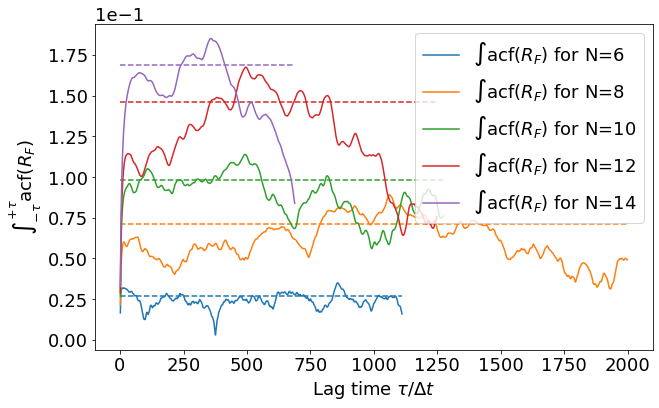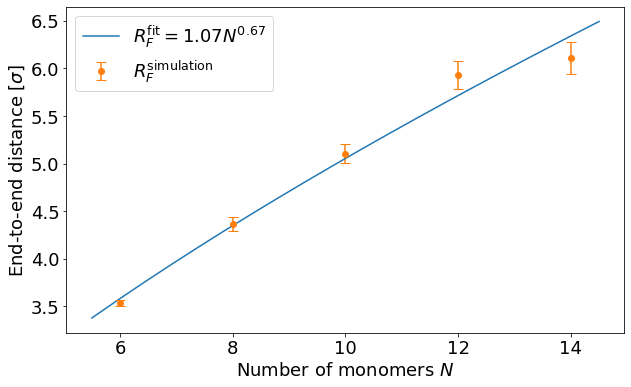Plot the radius of gyration $R_g$ of the polymer as a function of the number of monomers. What relation do you observe?

The radius of gyration follows the law $R_g = c_g N^\nu$ with $c_g$ a constant and $\nu$ the Flory exponent.

In :
rg_summary = standard_error_mean_autocorrelation(rg_results, r'\operatorname{acf}(R_g)')
rg_exponent, rg_prefactor = np.polyfit(np.log(N_MONOMERS), np.log(rg_summary[:, 0]), 1)
rg_prefactor = np.exp(rg_prefactor)

fig = plt.figure(figsize=(10, 6))
x = np.linspace(min(N_MONOMERS) - 0.5, max(N_MONOMERS) + 0.5, 20)
plt.plot(x, rg_prefactor * x**rg_exponent, '-',
label=rf'$R_g^{{\mathrm{{fit}}}} = {rg_prefactor:.2f} N^{{{rg_exponent:.2f}}}$')
plt.errorbar(N_MONOMERS, rg_summary[:, 0],
yerr=rg_summary[:, 1],
ls='', marker='o', capsize=5, capthick=1,
label=r'$R_g^{\mathrm{simulation}}$')
plt.xlabel('Number of monomers $N$')
plt.ylabel('Radius of gyration [$\sigma$]')
plt.legend()
plt.show()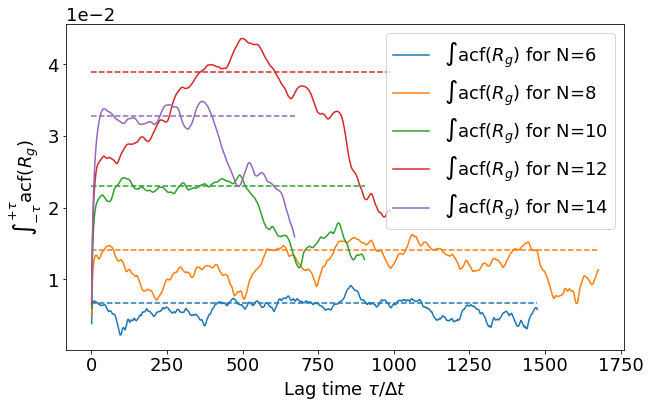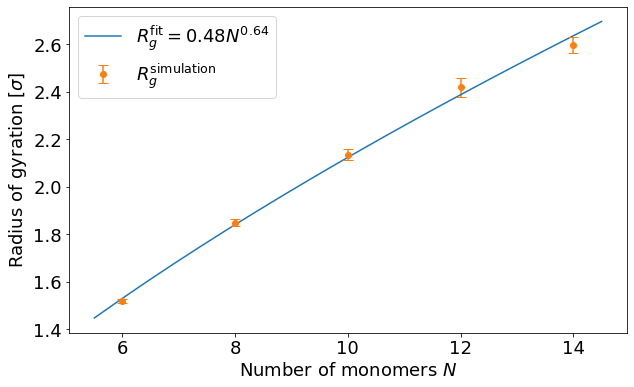For an ideal polymer:

$$\frac{R_F^2}{R_g^2} = 6$$
In :
rf2_rg2_ratio = rf_summary[:, 0]**2 / rg_summary[:, 0]**2
print(np.around(rf2_rg2_ratio, 1))

[5.4 5.6 5.7 6.  5.5]


Plot the hydrodynamic radius $R_h$ of the polymers as a function of the number of monomers. What relation do you observe?

The hydrodynamic radius can be calculated via the Stokes radius, i.e. the radius of a sphere that diffuses at the same rate as the polymer. An approximative formula is $R_h \approx c_h N^{1/3}$ with $c_h$ a constant.

In :
rh_summary = standard_error_mean_autocorrelation(rh_results, r'\operatorname{acf}(R_h)')
rh_exponent, rh_prefactor = np.polyfit(np.log(N_MONOMERS), np.log(rh_summary[:, 0]), 1)
rh_prefactor = np.exp(rh_prefactor)

fig = plt.figure(figsize=(10, 6))
x = np.linspace(min(N_MONOMERS) - 0.5, max(N_MONOMERS) + 0.5, 20)
plt.plot(x, rh_prefactor * x**rh_exponent, '-',
label=rf'$R_h^{{\mathrm{{fit}}}} = {rh_prefactor:.2f} N^{{{rh_exponent:.2f}}}$')
plt.errorbar(N_MONOMERS, rh_summary[:, 0],
yerr=rh_summary[:, 1],
ls='', marker='o', capsize=5, capthick=1,
label=r'$R_h^{\mathrm{simulation}}$')
plt.xlabel('Number of monomers $N$')
plt.ylabel('Hydrodynamic radius [$\sigma$]')
plt.legend()
plt.show()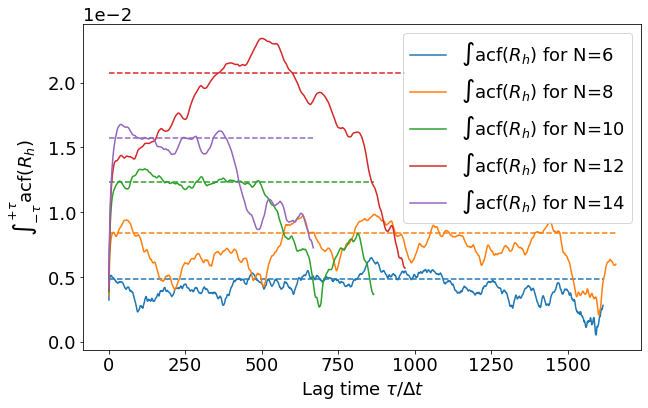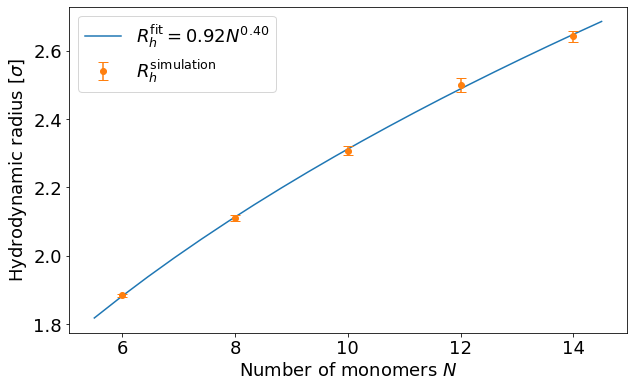#### 3.2 Diffusion coefficient using the MSD method¶

Calculate the diffusion coefficient of the polymers using the mean-squared displacement.

Recalling that for large $t$ the diffusion coefficient can be expressed as:

$$6D = \lim_{t\to\infty} \frac{\partial \operatorname{MSD}(t)}{\partial t}$$

which is simply the slope of the MSD in the diffusive mode.

In :
# cutoff for the diffusive regime (approximative)
tau_f_index = 40
# cutoff for the data series (larger lag times have larger variance due to undersampling)
tau_max_index = 70

plt.figure(figsize=(10, 10))
plt.xlabel(r'$\tau$ [$\Delta t$]')
plt.ylabel(r'MSD [$\sigma^2$]')
for index, (tau, msd) in enumerate(zip(com_pos_tau_results, com_pos_msd_results)):
plt.loglog(tau[1:120], msd[1:120], label=f'N={N_MONOMERS[index]}')
plt.loglog(2 * [tau[tau_f_index]], [0, np.max(com_pos_msd_results)], '-', color='black')
plt.text(tau[tau_f_index], np.max(com_pos_msd_results), r'$\tau_{f}$')
plt.loglog(2 * [tau[tau_max_index]], [0, np.max(com_pos_msd_results)], '-', color='black')
plt.text(tau[tau_max_index], np.max(com_pos_msd_results), r'$\tau_{max}$')
plt.legend()
plt.show()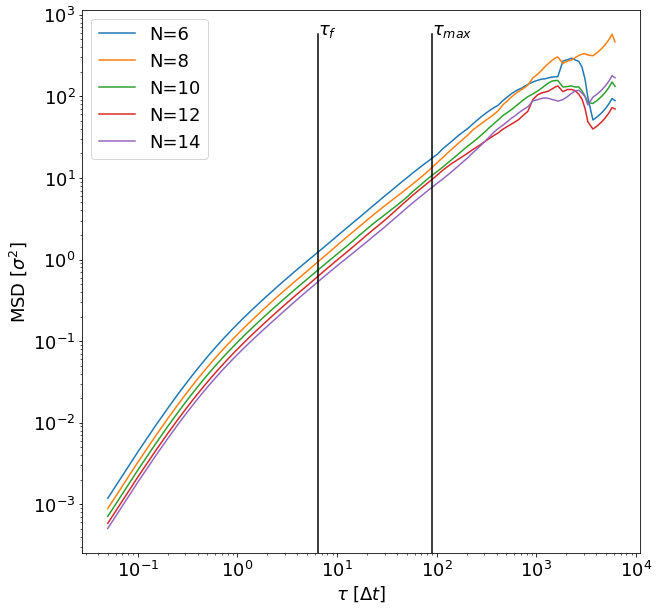In :
diffusion_msd = np.zeros(len(N_MONOMERS))
plt.figure(figsize=(10, 8))
weights = com_pos_cor.sample_sizes()
for index, (tau, msd) in enumerate(zip(com_pos_tau_results, com_pos_msd_results)):
a, b = np.polyfit(tau[tau_f_index:tau_max_index], msd[tau_f_index:tau_max_index],
1, w=weights[tau_f_index:tau_max_index])
x = np.array([tau, tau[tau_max_index - 1]])
p = plt.plot(x, a * x + b, '-')
plt.plot(tau[1:tau_max_index], msd[1:tau_max_index], 'o', color=p.get_color(),
label=rf'$N=${N_MONOMERS[index]}')
diffusion_msd[index] = a / 6
plt.xlabel(r'$\tau$ [$\Delta t$]')
plt.ylabel(r'MSD [$\sigma^2$]')
plt.legend()
plt.show()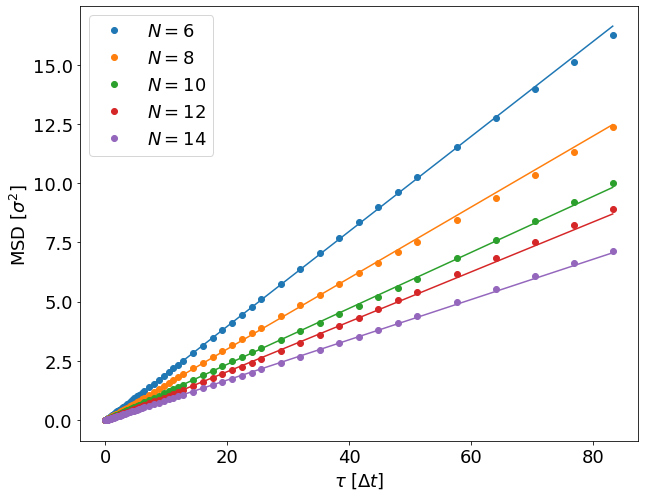Plot the dependence of the diffusion coefficient on the hydrodynamic radius.

Recalling the formula for the diffusion coefficient of a short polymer in the Kirkwood–Zimm model:

$$D = \frac{D_0}{N} + \frac{k_B T}{6 \pi \eta} \left\langle \frac{1}{R_h} \right\rangle$$

where $\eta$ is the fluid viscosity and $D_0 = k_BT\gamma^{-1}$ the monomer diffusion coefficient, with $\gamma$ the fluid friction coefficient. For the Rouse regime (implicit solvent), the second term disappears.

Hint:

• for the Rouse regime, use $D = \alpha N^{-1}$ and solve for $\alpha$
• for the Zimm regime, use $D = \alpha_1 N^{-1} + \alpha_2 N^{-\beta}$ with rh_exponent for $\beta$ and solve for $\alpha_1, \alpha_2$
In :
fig = plt.figure(figsize=(10, 6))
x, y, label, popt_msd = fitting_polymer_theory(POLYMER_MODEL, N_MONOMERS, diffusion_msd, rh_exponent)
plt.plot(x, y, '-', label=label)
plt.plot(N_MONOMERS, diffusion_msd, 'o', label=r'$D^{\mathrm{simulation}}$')
plt.xlabel('Number of monomers $N$')
plt.ylabel(r'Diffusion coefficient [$\sigma^2/t$]')
plt.ticklabel_format(axis='y', style='sci', scilimits=(0, 0))
plt.legend()
plt.show()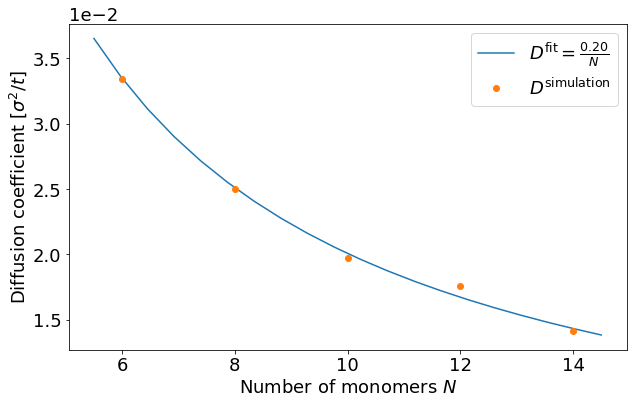#### 3.3 Diffusion coefficient using the Green–Kubo method¶

Plot the autocorrelation function and check that the decay is roughly exponential.

Hint: use $D = \alpha e^{-\beta \tau}$ and solve for $\alpha, \beta$. You can leave out the first data point in the ACF if necessary, and limit the fit to the stable region in the first 20 data points.

In :
def exponential(x, a, b):
return a * np.exp(-b * x)

fig = plt.figure(figsize=(10, 8))
for N, tau, acf in zip(N_MONOMERS, com_vel_tau_results, com_vel_acf_results):
popt, _ = scipy.optimize.curve_fit(exponential, tau[:20], acf[:20])
x = np.linspace(tau, tau[20 - 1], 100)
p = plt.plot(x, exponential(x, *popt), '-')
plt.plot(tau[:20], acf[:20], 'o',
color=p.get_color(), label=rf'$R(\tau)$ for N = {N}')
plt.xlabel(r'$\tau$')
plt.ylabel('Autocorrelation function')
plt.legend()
plt.show()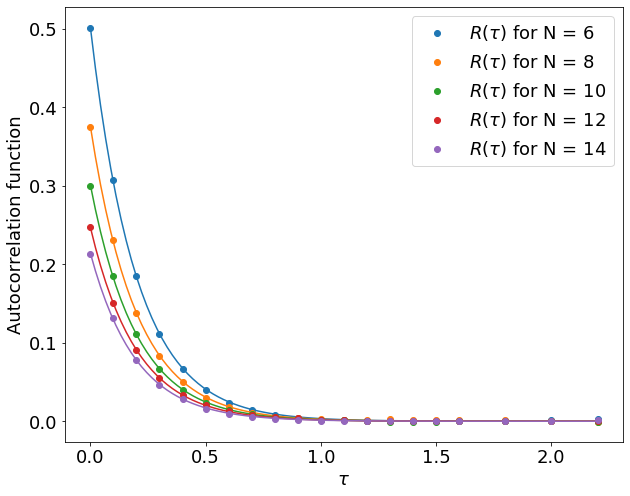The Green–Kubo integral for the diffusion coefficient take the following form:

$$D = \frac{1}{3} \int_0^{+\infty} \left<\vec{v_c}(\tau)\cdot\vec{v_c}(0)\right>\, \mathrm{d}\tau$$

Since our simulation is finite in time, we need to integrate up until $\tau_{\mathrm{int}}$. To find the optimal value of $\tau_{\mathrm{int}}$, plot the integral as a function of $\tau_{\mathrm{int}}$ until you see a plateau. This plateau is usually followed by strong oscillations due to low statistics in the long time tail of the autocorrelation function.

In :
diffusion_gk = []
fig = plt.figure(figsize=(10, 6))
for N, tau, acf in zip(N_MONOMERS, com_vel_tau_results, com_vel_acf_results):
x = np.arange(2, 28)
y = [1 / 3 * np.trapz(acf[:j], tau[:j]) for j in x]
plt.plot(tau[x], y, label=rf'$D(\tau_{{\mathrm{{int}}}})$ for $N = {N}$')
diffusion_gk.append(np.mean(y[10:]))
plt.xlabel(r'$\tau_{\mathrm{int}}$')
plt.ylabel(r'$\frac{1}{3} \int_{\tau=0}^{\tau_{\mathrm{int}}} \left<\vec{v_c}(\tau)\cdot\vec{v_c}(0)\right>\, \mathrm{d}\tau$')
plt.ticklabel_format(axis='y', style='sci', scilimits=(0, 0))
plt.legend()
plt.show()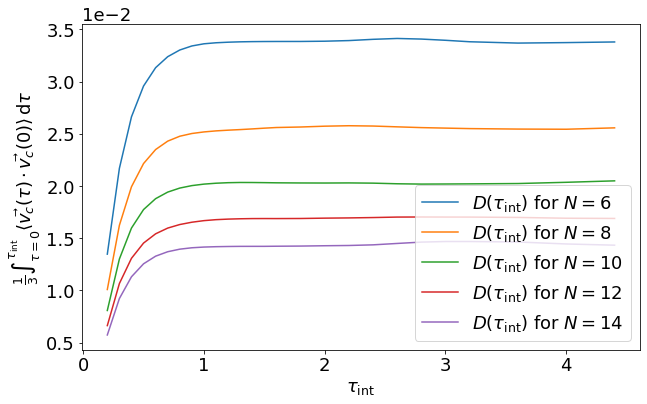Plot the dependence of the diffusion coefficient on the hydrodynamic radius.

In :
fig = plt.figure(figsize=(10, 8))
x, y, label, popt_gk = fitting_polymer_theory(POLYMER_MODEL, N_MONOMERS, diffusion_gk, rh_exponent)
plt.plot(x, y, '-', label=label)
plt.plot(N_MONOMERS, diffusion_gk, 'o', label=r'$D^{\mathrm{simulation}}$')
plt.xlabel('Number of monomers $N$')
plt.ylabel(r'Diffusion coefficient [$\sigma^2/t$]')
plt.ticklabel_format(axis='y', style='sci', scilimits=(0, 0))
plt.legend()
plt.show()